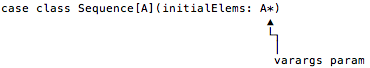How To Make Sequence Work as a Single Generator in a `for` Expression

This is a page from my book, Functional Programming, Simplified

Getting Sequence to work as a generator in a simple for loop was cool, but does adding foreach let Sequence also work when I add yield? Let’s see.

When I paste this code into the REPL:

val ints = Sequence(1,2,3)

for {
i <- ints
} yield i*2

I see this error message:

scala> for {
|     i <- ints
| } yield i*2
<console>:15: error: value map is not a member of Sequence[Int]
i <- ints
^

Sadly, Sequence won’t currently work with for/yield, but again the REPL tells us why:

error: value map is not a member of Sequence[Int]

That error tells us that Sequence needs a map method for this to work. Great — let’s create one.

Adding a `map` method to Sequence

Again I’m going to cheat to create a simple solution, this time using ArrayBuffer’s map method inside Sequence’s map method:

def map[B](f: A => B): Sequence[B] = {
val abMap: ArrayBuffer[B] = elems.map(f)
Sequence(abMap: _*)
}

This map method does the following:

• It takes a function input parameter that transforms a type A to a type B.
• When it’s finished, map returns a Sequence[B].
• In the first line of the function I show abMap: ArrayBuffer[B] to be clear that elems.map(f) returns an ArrayBuffer. As usual, showing the type isn’t necessary, but I think it helps to make this step clear.
• In the second line inside the function I use the :_* syntax to create a new Sequence and return it.

About the :_* syntax

If you haven’t seen the abMap: _* syntax before, the :_* part of the code is a way to adapt a collection to work with a varargs parameter. Recall that the Sequence constructor is defined to take a varags parameter:For more information on this syntax, see my information on Scala’s missing splat operator.

The complete Sequence class

This is what the Sequence class looks like when I add the map method to it:

case class Sequence[A](initialElems: A*) {

private val elems = scala.collection.mutable.ArrayBuffer[A]()

// initialize
elems ++= initialElems

def map[B](f: A => B): Sequence[B] = {
val abMap = elems.map(f)
new Sequence(abMap: _*)
}

def foreach(block: A => Unit): Unit = {
elems.foreach(block)
}

}

Does for/yield work now?

Now when I go back and try to use the for/yield expression I showed earlier, I find that it compiles and runs just fine:

scala> val ints = Sequence(1,2,3)
ints: Sequence[Int] = Sequence(WrappedArray(1, 2, 3))

scala> for {
|     i <- ints
| } yield i*2
res0: Sequence[Int] = Sequence(ArrayBuffer(2, 4, 6))

An important point

One point I need to make clear is that this for/yield expression works solely because of the map method; it has nothing to do with the foreach method.

You can demonstrate this in at least two ways. First, if you remove the foreach method from the Sequence class you’ll see that this for expression still works.

Second, if you create a little test class with this code in it, and then compile it with scalac -Xprint:parse, you’ll see that the Scala compiler converts this for expression:

for {
i <- ints
} yield i*2

into this map expression:

ints.map(((i) => i.\$times(2)))

To be very clear, creating a foreach in Sequence enables this for loop:

for (i <- ints) println(i)

and defining a map method in Sequence enables this for expression:

for {
i <- ints
} yield i*2

Summary

I can summarize what I accomplished in this lesson and the previous lesson with these lines of code:

// (1) works because `foreach` is defined
for (p <- peeps) println(p)

// (2) `yield` works because `map` is defined
val res: Sequence[Int] = for {
i <- ints
} yield i * 2
res.foreach(println)  // verify the result

What’s next?

This is a good start. Next up, I’ll modify Sequence so I can use it with filtering clauses in for expressions.